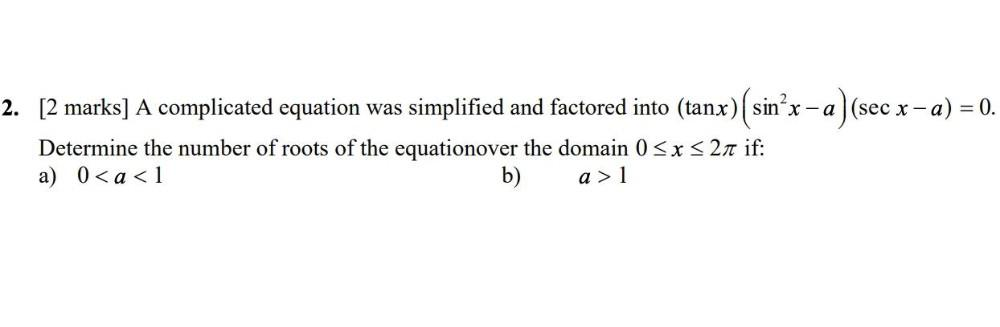Question:

# A complicated equation was simplified and factored into (tanx) (sin²x – a ) (sec x - a) = 0. Determine the number of roots of the equation over the domain 0 <= x <= 2π if: a) 0< a < 1 b) a > 1A complicated equation was simplified and factored into (tanx) (sin²x – a ) (sec x - a) = 0. Determine the number of roots of the equation over the domain 0 <= x <= 2π if: a) 0< a < 1 b) a > 1